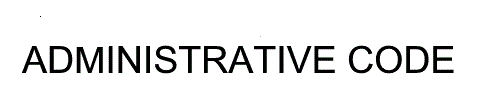TITLE 35: ENVIRONMENTAL PROTECTION SUBTITLE H: NOISE CHAPTER I: ILLINOIS POLLUTION CONTROL BOARD PART 910 MEASUREMENT PROCEDURES FOR THE ENFORCEMENT OF 35 ILL. ADM. CODE 900 & 901 SECTION 910.107 MEASUREMENT TECHNIQUES FOR HIGHLY IMPULSIVE SOUND UNDER 35 ILL. ADM. CODE 901.104.   Section 910.107  Measurement Techniques for Highly Impulsive Sound Under 35 Ill. Adm. Code 901.104.   a)         Measurement of highly impulsive sound under 35 Ill. Adm. Code 901.104 can be made using two distinct and equally valid ways specified in subsections (b) and (c), namely the general method and the controlled test method.    b)          General Method:  The general method is to measure the 1-hour, A-weighted Leq (not the octave-band or ⅓ octave-band levels) using essentially one of the two procedures described in Sections 910.105 and 910.106.   1)         The procedure using small blocks of time to collect data is as follows:   A)       Divide the hour interval into small blocks of time and measure the A-weighted Leq for each of these blocks.  Leq is  measured for the entire hour but data collection is inhibited whenever a short-term background transient sound occurs.   B)        The duration of each block is held constant during the hour.  This duration in seconds divides exactly into 900 and is neither greater than 100 seconds nor less than 10 seconds.   C)        Discard the data for any block corrupted by one or more short-term background ambient sounds.   2)         Correction for the Long-Term Background Ambient Sound.  Correct the raw 1-hour Leq for long-term ambient sound using the procedures of Sections 910.105 and 910.106 to determine an A-weighted, 1-hour, background-ambient-corrected Leq for the highly impulsive property-line noise source under study.    c)         Controlled Test Method   1)         General Measurement Description   A)       The sound exposure per impulse from each separate individual impulsive source is measured.   B)        The total sound exposure per hour from each source is the sound exposure per event multiplied by the number of events per hour.   C)        The grand total sound exposure (SE) per hour is the sum of the sound exposures per hour from each of the separate individual sources.    D)       The reported SEL is obtained from the grand total sound exposure (SE) per hour using the following:   SEL = 10 log (SE) + 94                                   [Equation  7]   E)        The equivalent level, Leq, corresponding to a SEL measured or predicted for one hour (3600 seconds) is given by:   Leq = SEL - 10 log (3600)                                [Equation  8]   2)         Determination of Sound Exposure Per Event   A)       Determine the sound exposure per event from each, separate, individual source by measuring the total A-weighted sound exposure for about 10 repetitions of the source.  This set of about 10 measurements may be performed continuously over a short period of time, or over a discontinuous set of measurement periods.  In either case, the total measurement duration must be less than 100 seconds.   B)         The separate, individual property-line noise source measurements collected under subsection (a) must be free of any short-term ambient sounds.  If any short-term background transient sounds occur during these measurements, repeat  the measurements until data, free of any corrupting short-term background ambient sounds, are obtained.    C)         Correct the total measured A-weighted sound exposure for the group of about 10 repetitions for long-term background ambient by subtracting the A-weighted long-term background ambient sound exposure, which is the long-term A-weighted background ambient sound exposure per second multiplied by the number of seconds used to measure the several source repetitions.    D)       A-weighted sound exposure per event is the total corrected sound exposure divided by the number of source repetitions measured.   E)        Measure the long-term background ambient for a short time, at least 30 seconds, as near in time to the source measurements as possible, but within ½ hour.  The total A-weighted long-term background ambient sound exposure per second is the total measured long-term background ambient sound exposure divided by the number of seconds of background ambient measurement.   F)        There must be no short-term background ambient sounds present during the measurement of the long-term background ambient.  If any short-term background transient sounds occur during these measurements, repeat the measurements until long-term background ambient measurement data, free of any corrupting short-term background ambient sound, are obtained.   (Source:  Amended at 42 Ill. Reg. 20487, effective November 1, 2018)